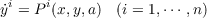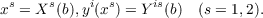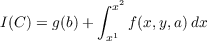#### Vol. 17, No. 2, 1966

 Download this articleFor screen For printingRecent Issues Vol. 323: 1  2 Vol. 322: 1  2 Vol. 321: 1  2 Vol. 320: 1  2 Vol. 319: 1  2 Vol. 318: 1  2 Vol. 317: 1  2 Vol. 316: 1  2Online Archive Volume: Issue:The Journal Subscriptions Editorial Board Officers Contacts Submission Guidelines Submission Form Policies for Authors ISSN: 1945-5844 (e-only) ISSN: 0030-8730 (print) Special Issues Author Index To Appear Other MSP Journals
Sufficient conditions for an optimal control problem in the calculus of variations

### Edwin Haena Mookini

Vol. 17 (1966), No. 2, 263–277
##### Abstract

An arc C is a collection of parameters bρ (ρ = 1,,r) on an open set B and sets of functions yi(x), ah(x)(i = 1,,n;h = 1,,m) defined on an interval x1 x x2 with yi(x) continuous and i(x), ah(x) piecewise continuous. The arc is admissible if it satisfies the differential equationson x1 x x2 and the end conditionsThe dot denotes differentiation with respect to x. The problem at hand is to find in a class of admissible arcs C, an arc C0, which minimizes the integralwhere P(x,y,a) and f(x,y,a) are assumed to be class C′′ for (x,y,a) in an open set R while g(b), Xs(b), Y is(b) are of class C′′ on B. Under the added assumption that P(x,y,a) is Lipschitzian in y and a, the indirect method of Hestenes is used to prove that the necessary conditions for relative minima of the problem above, strengthened in the usual manner, yield a set of sufficient conditions. This problem differs from that of Pontryagin in the choice of (x,y,a) to lie in an open set.

Primary: 93.40
Secondary: 49.00
##### Milestones